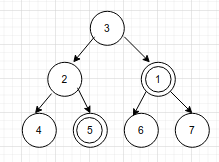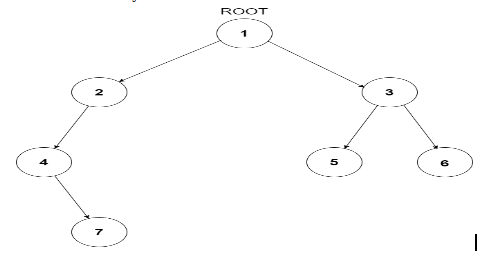# Least Common Ancestor

Posted: 14 Jan, 2021
Difficulty: Moderate

## PROBLEM STATEMENT

#### For example look at the tree below, the LCA of node 1 and 5 is 3.#### Note :

``````You have to return the deepest node which has both x, y as its descendants.
There may be cases where one of u or v is not present in the tree. In those cases, the reference provided to u or v will be NULL.
``````
##### Input Format :
``````The first line of the input contains a single integer T, representing the number of test cases.

The first and only line of each test case contains the values of the nodes of the tree in the level order form ( -1 for NULL node) Refer to the example for further clarification.
``````

Example: Consider the binary tree##### The input of the tree shown in the above image will look like:
``````1 2 3 4 -1 5 6 -1 7 -1 -1 -1 -1 -1 -1

Explanation :
Level 1 :
The root node of the tree is 1

Level 2 :
Left child of 1 = 2
Right child of 1 = 3

Level 3 :
Left child of 2 = 4
Right child of 2 = null (-1)
Left child of 3 = 5
Right child of 3 = 6

Level 4 :
Left child of 4 = null (-1)
Right child of 4 = 7
Left child of 5 = null (-1)
Right child of 5 = null (-1)
Left child of 6 = null (-1)
Right child of 6 = null (-1)

Level 5 :
Left child of 7 = null (-1)
Right child of 7 = null (-1)

The first not-null node (of the previous level) is treated as the parent of the first two nodes of the current level. The second not-null node (of the previous level) is treated as the parent node for the next two nodes of the current level and so on. The input ends when all nodes at the last level are null (-1).
``````
##### Output Format :
``````For each test case, print the LCA of the given nodes in a separate line.
``````
##### Note :
``````You do not need to print anything. It has already been taken care of. Just implement the given function.
``````
##### Constraints :
``````1 <= T <= 50
1 <= N <= 10^4
1 <= nodeVal <= 10^9

Time Limit: 1 sec
``````Approach 1
• We know that the binary tree is a recursive structure, so we can use recursion to find the answer.
• Let getLCA() be the recursive function which takes the root of the tree and the two nodes u and v as a parameter and returns LCA of the nodes.
• If the current subtree contains both the nodes, then the function result is their LCA.
• Now if the root is equal to u or v, then root is the answer.
• Let ‘left’ store the result of LCA in the left subtree of the current node. If both the nodes are not present, then return NULL
• Let ‘right’ store the result LCA  in the right subtree. If both the nodes are not present, then return NULL.
• Now if both left and right are NULL then return NULL
• If left != NULL and right != NULL, then return root.
• If left = NULL, then return right else return left.